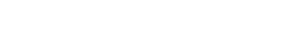# Two Way Table / Two Way Frequency Tables

## What is a Two Way Table?

A two way table is a way to display frequencies or relative frequencies for two categorical variables. One category is represented by rows and a second category is represented by columns.

### Example

Sixty people (30 men and 30 women) were asked what type of movie they would prefer to watch and the following responses were recorded:

• 6 men preferred rom-coms.
• 16 men preferred action movies.
• 8 men preferred horror movies.
• 12 women preferred rom-coms.
• 14 women preferred action movies.
• 4 women preferred horror movies.

The information collected was used to build the following two way table:Two way table showing movie preferences.

The entries in the table are counts; this type of table is called a two way frequency table. The table has several features:

• Categories are labeled in the left column and top row.
• The counts are placed in the center of the table.
• Totals appear at the end of each row and column.
• A sum of all counts (a grand total) is placed at the bottom right.
• The totals in the right column and bottom row are called marginal distributions (excluding the grand total).
• The entries in the center of the table (everything except the marginal distributions) are called joint frequencies.

## Two Way Relative Frequency Table

Instead of displaying counts in the table, you could show relative frequencies. This is the same two way relative frequency table (decimals, percents, or ratios) displayed instead of counts:To convert counts into relative frequencies, divide the count by the total number of items. In the above table, the first count is for men / Rom-com (count=6), so 6/60 = 0.1.
The totals in the right column and bottom row are, like the two way frequency table, called marginal distributions. However, the entries in the center of the table are called conditional frequencies or conditional distributions.

Two way frequency tables are sometimes called contingency tables, but that term is usually only used once you get into more advanced statistics that involves research and hypothesis testing.

Check out our YouTube channel for hundreds of elementary statistics and probability how-to videos!

## References

Agresti A. (1990) Categorical Data Analysis. John Wiley and Sons, New York.
Kotz, S.; et al., eds. (2006), Encyclopedia of Statistical Sciences, Wiley.
Levine, D. (2014). Even You Can Learn Statistics and Analytics: An Easy to Understand Guide to Statistics and Analytics 3rd Edition. Pearson FT Press

CITE THIS AS:
Stephanie Glen. "Two Way Table / Two Way Frequency Tables" From StatisticsHowTo.com: Elementary Statistics for the rest of us! https://www.statisticshowto.com/two-way-table/
---------------------------------------------------------------------------Need help with a homework or test question? With Chegg Study, you can get step-by-step solutions to your questions from an expert in the field. Your first 30 minutes with a Chegg tutor is free!